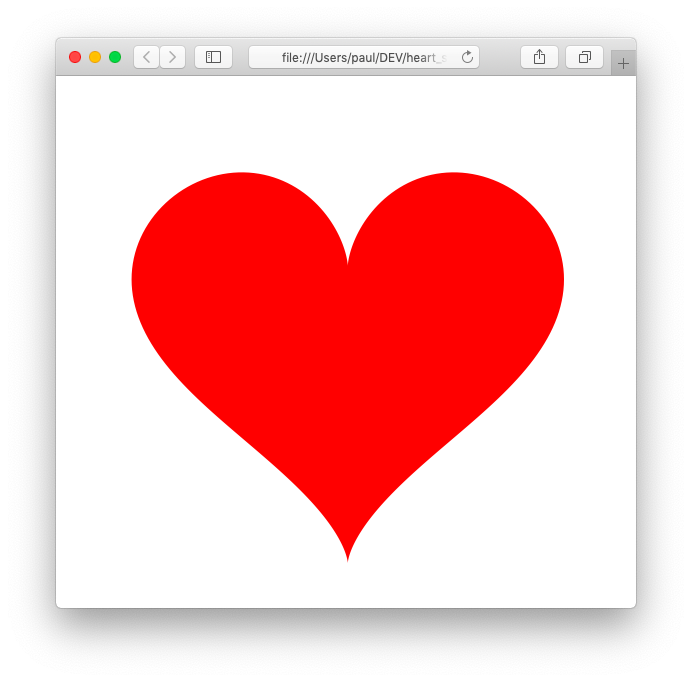# C++17 - Draw a Valentine's Day heart shape

## Posted on February 14, 2019 by Paul

What could be more romantic than to generate a heart shape with C++17 on Valentine’s Day ?In order to generate the above shape we can use the parametric form of the outer curve:

$x(t) = 16 \cdot sin(t)^3;$ $y(t) = 13 \cdot cos(t) - 5 \cdot cos(2 \cdot t) - 2 \cdot cos(3 \cdot t) - cos(4 \cdot t);$

where

$t \in [0, 2 \cdot \pi]$

Using the above equations, we can generate the x, y points of the heart curve. In order to actually draw the points, we can use a third party graphics library or, if we want a simpler, self-contained code, we can write the points as an image. In this article, I will show you how to write the points as an SVG image embedded in a html page. The advantage of this approach is that you will be able to see the generated shape in any modern web browser. If you prefer to generate a BMP image check my previous article.

We want to be able to write something like this:

Please note, that line 18 from the above code uses structured bindings declaration that requires a C++17 compatible compiler. Basically, we use the vx and vy references as aliases instead of the more clunky std::tuple syntax.

Let’s write the code that generates the curve points. We’ll divide the $$[0, 2 \cdot \pi]$$ interval in equal $$2 \cdot \pi / no\_pieces$$ pieces.

Once we’ve generated the curve points, we need to flip the Y coordinates because, like most image formats, SVG has the Y axis pointing down. We can do this in the main function:

Next, we’ll write the function that will save the shape as an SVG image embedded in a html page. I’ve commented the function to make it easier to understand. If you want to learn more about generating SVG shapes check this MDN tutorial.

The above function will write something like this, please note that the numeric output was truncated in order to fit on the page:

Finally, we can call the write_html_svg from main like this:

I’ve tested the code with GCC 8, Clang 7 and MSVC 2017. Here is how you can compile the code with the above compilers (use the command that matches your compiler):

This is how you can compile and run the code on macOS (or Linux):

On Windows, with Visual Studio installed, open a Visual Studio Command Prompt and do something like this (I assume that the code is in the C:\Tests folder):

Once the heart.html file is generated, open it in your browser and you should see something similar with the image from the beginning of this article.

You can find the complete source code on the GitHub repository for this article.